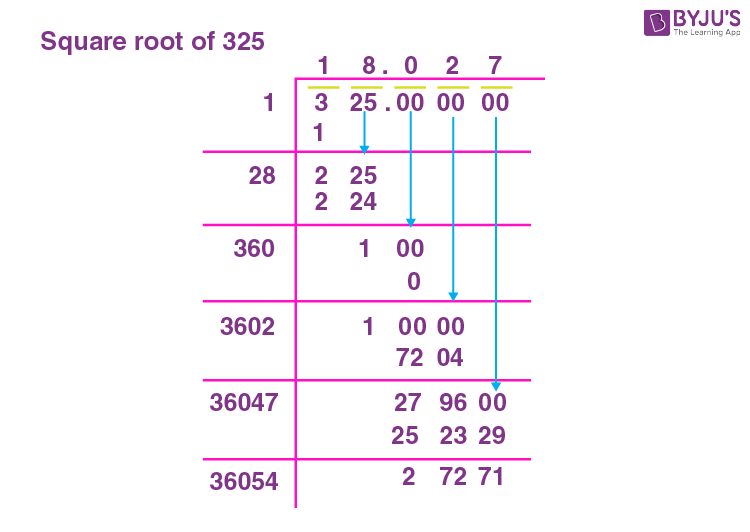# Square Root of 325

The square root of 325 is irrational. The approximate value of the square root of 325 is 18.03.

If 18.03 is the square root of 325, it means that if 18.03 is multiplied by 18.03, then the resultant number is 325 (324.72, rounded off to get 325). Here 18.03 is the square root of 325 (324.72). If an integer is multiplied by itself, then the resultant is termed the perfect square number and the integer multiplied by itself is the perfect square root. Representing it with Mathematical notation would be “ n × n = r”, where r is the perfect square number and “n” is its square root. Note that “n” can be an integer. The methods to find “n” are either by prime factorisation method, long division method or repeated subtraction method. You can refer to the Finding Square root with Example.

Note the Following:

• The Square root of 325 = 2√325 = √325 where ‘√’ is the radical, and 325 is the radicand and 2 is the index.
• Exponential Form of Square root of 325 = 3251/2
• Solution for √325 ≈18.02
• The square root of 325 is Irrational = True

## What is the Square root of 325?

The square root of 325 is18.02, as the square root of 325 is irrational.

 √325 =18.03

## How to Find the Square root of 325?

There are three methods to find the Square root of 325

• Prime Factorisation method
• Long Division method
• Repeated Subtraction method

### Square root of 325 by Prime Factorisation Method

In the prime factorization method, 325 is divided by prime factors, starting from 5.

The division on 325 using prime factors is given below;

 5 325 5 65 13 13 × 1

The prime factors are 5, 5 and 13. Since this is a square root, two 5’s can be removed from within the root as 5. i,e √325 = √(5× 5 × 13) = 5√13.

### Square root of 325 by Long Division Method

To understand the long division method, follow the below-mentioned detailed steps;

Step 1: Group the given number into pairs.

Step 2: Divide the first pair, using the divisor such that the product is less than or equal to.

Step 3: Continue the division by bringing the next pair

Step 4: The first divisor in the next division to be (Divisor of first division + quotient of first division) such that:

d1 × 1 =

d2 × 2 =

d3 x 3 =

And so on.

Step 5: If the division is complete with the remainder as zero, then the quotient will be the square root. Else the number is not a perfect square number.

Applying the above steps to 325.

Pairing 1 and 49.

The squares are:

1 × 1 = 1

2 × 2 = 4Hence 325 does not have a perfect square. Therefore, 325 is an irrational number whose root lies between 18 and 19.

### Square root of 325 by Repeated Subtraction Method.

When 325 is subtracted by one, and the resultant by 3 and its resultant by 5. Continuing the subtraction repeatedly by odd numbers is the repeated subtraction method of finding the square root. When this process results in zero at any step, then the step is the perfect square root of 325, else the number 325 is irrational.

The table below shows repeated subtraction for the given number 325.

 Step 1 325 – 1 = 324 Step 2 324 – 3 = 321 Step 3 321 – 5 = 316 Step 4 316 – 7 = 309 Step 5 309 – 9 = 300 Step 6 300 – 11 = 289 Step 7 289 – 13 = 276 Step 8 276 – 15 = 261 Step 9 261 – 17 = 244 Step 10 244 – 19 = 225 Step 11 225 – 21 = 204 Step 12 204 – 23 = 181 Step 13 181 – 25 = 156 Step 14 156 – 27 = 129 Step 15 129 – 29 = 100 Step 16 100 – 31 = 69 Step 17 69 – 33 = 36 Step 18 36 – 35 = 1 Step 19 1 – 37 = -36

Since the difference does not become zero, 325 is not a perfect square number and hence it does not have a perfect square root. The root lies between the numbers 18 and 19, resulting in a decimal root.

## Video Lessons

### Visualising square roots### Finding Square roots## Solved Examples

1. Does 325 have a perfect square root?

Solution: No. 325 does not have a perfect square root.

2. What is the square of 325?

Solution: 325 square is 105625.

## Frequently Asked Questions on Square root of 325

### What is the Square root of 325?

The Square root of 325 is 18.03.

### 325 is a perfect square number. True or False?

False. 325 is not a perfect square number.

### What are the prime factors of 325?

The prime factors of 325 are 5 and 13.

### Simplify √325?

√325 = √(5 × 5 × 13) = 5√13, no further simplification can be done.

### The square root of 325 is an irrational number. True or False?

True. The square root of 325 is an irrational number.